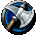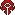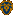## FANDOM

106,204 PagesThis article is a guild progression information page for the Scarlet Crusade US realm (server). The contents herein are entirely player made and in no way represent official World of Warcraft history or occurrences which are accurate for all realms. This page includes a list of guilds that have accomplished a particular task (usually by defeating a major boss). The list should not be taken to be 100% accurate, as it only contains data that has been submitted/added by users of the community. Thus, if you have information you can add, and contribute to the list, it will become more complete.

You can find the new page at Guild Progress for BC Instances -Zeeps

### Typical Instance Order and Tier Levels

Tier 4: Tier 5: Tier 6: Tier 6 Equivalent & Higher Gear

### Guild Progression - BC

Gruul's Lair Magtheridon's Lair Serpentshrine Cavern The Eye Battle for Mount Hyjal Black Temple Sunwell Plateau
Guild HKM G M H Lu MT FLK Le V A VR HAS KS RW An K Az Ar HWN S GB SoA TG RoS MS IC IllidanAscent X X X X X X X X X X X X X X X X X X X X X X X X X X XEternal X X X X X X X X X X X X X X X X X X X X X X X X X X XSynergy was Soulstoned X X X X X X X X X X X X X X X X X X X X X X X X X X XArchon X X X X X X X X X X X X X X X X X X X X X X X XScarlet Aristocracy X X X X X X X X X X X X X X X X X X X X XAbsolution X X X X X X X X X X X X X X X X X X X XThe Green Crusade X X X X X X X X X X X X X X X X XMischief X X X X X X X X X X X XRaid Awesome X X X X X X X X X X X XEternal Balance X X X X X X X X X XTempest / Meleestorm X X X X X X X X X X XShadowfyre X X X X X X X X XAngst X X X X X X X X X XLegio Fidelis X X X X X X X XImmortalis X X X X X X X XSaga X X X X X XThe Dark Company X X X X X XLok Tar Ogar X X X X XVindictive X X X X X X XThe Redeemed X X X X XThe Fallen Skulls X X X XThe Order of Mjolnir X X X XThe Reborn X X X XAegis Hestia X X Xguerilla X X XTriarii X XExertius of Obscurum X XPraetorian Knights X X X X X X X X X X X X X X X X X X X X X X X X X XMalice X XLegacy of Legends X XThe Foot Clan X X

Updated and Managed by: Zeeps/Zeta of Tempest or Voodu of Absolution
Last Updated: 2/13/2008 at 6:44pm CST

Order:
• Abomination Wing
• Deathknight Wing
• Plague Wing
• Spider Wing
• Frostwyrm Lair

Rank is determined by total number of bosses killed (count) with the date of the last kill used as a tie breaker.

## General Raid Progression (AQ40/BWL/MC)

The below table lists the last boss of each instance that the following guilds have killed (as opposed to which boss they are currently working on). An instance is "Complete" when all bosses (including optional) have been downed.

* Ladies of Destiny / The Fel Watch / Twilights Legacy / The Gnomes of Destiny
** Mischief / Xukuth d'Oloth
*** Night Fire Dragons / The Fallen Skulls / The Forsaken Brotherhood
**** Eyes of the Dragon / Unchained / Sanguine Steel
***** Nocturnal / Arcadian Reign / Order of Tyr
****** Alliance Raid PUG - Raid Leader: Stacybeau of The Eternal Balance, Occurring after the release of World of Warcraft: The Burning Crusade

Community content is available under CC-BY-SA unless otherwise noted.